# Multiplying Decimals

In Mathematics, we may come across different types of numbers. Decimals are specific types of numbers, with a whole number and the fractional part separated by a decimal point. The dot that exists between the whole number and fractions part is called the decimal point.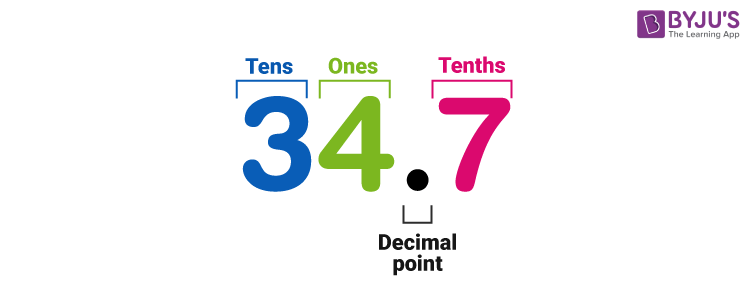Various arithmetic operations can be performed on these numbers, such as addition, subtraction, multiplication and division. In this article, you can learn about multiplying the decimals along with solved examples.

## How to Multiply Decimals

Multiplying Decimals is similar to multiplying whole numbers, except for the way we handle the decimal point. There are two different cases in dividing decimals, such as:

• Multiplying Decimals by Whole number
• Multiplying Decimal by Decimal Number

However, when we multiply decimal numbers, we may get a decimal or whole number. Let’s understand the division of these decimals in detail here.

Also, get a multiplying decimals calculator here.

## Multiplying Decimals by Whole Numbers

Multiplying decimal numbers by whole numbers can be done as we do the multiplication of whole numbers. In this case, we should only take care of the decimal point. However, the operation of whole numbers multiplying with decimals is shown in the below figure.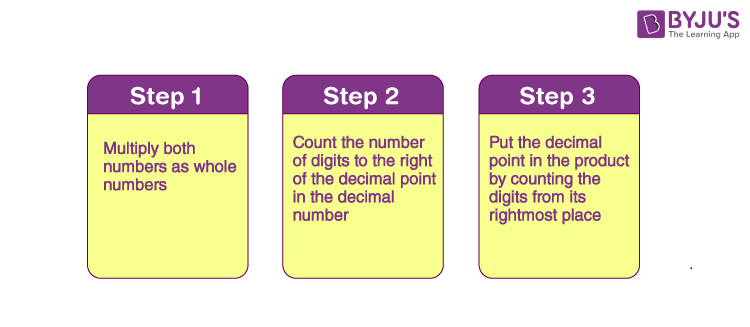The above process can be understood in a better way with the example given below.

Example: Multiply 4785.25 by 8

Solution:

Step 1:

Multiply the both numbers as whole numbers, i.e. we ignore the decimal point here and proceed with usual multiplication.

478525 × 8 = 3828200

Step 2:

Counting the number of digits to the right of the decimal point in the decimal number.

In this case, the required number of digits = 2

Step 3:

Put the decimal point in the product by counting the digits from the rightmost place.

As the total number of decimal places is 2, the resultant product must contain the decimal point after 2 digits from the rightmost place.

Therefore, 4785.25 × 8 = 38282.00 = 38282

## Multiplying Decimals by 10 100 and 1000

Let us find the multiplication of a decimal number by 10, 100 and 1000.

Consider 32.47 × 10 = 324.7. Thus, the digits in 32.47 and 324.7 are the same, i.e., 3, 2, 4, and 7, but the decimal point has shifted (towards the right) in the result. Therefore, in a decimal multiplication by 10, the decimal point will shift to the right by one place since 10 has only one zero over 1.

From the above example, we can say that the number and product digits will be the same in the multiplication of decimals by 10, 100 or 1000. However, the decimal point in the obtained product of the multiplication will shift towards the right by as many places as zeros over 1.

Let’s have a look at some examples of multiplication of decimal numbers by 10, 100, and 1000.

• 42.5 × 10 = 425
• 647.124 × 10 = 6471.24
• 218.19 × 100 = 21819
• 126.3 × 100 = 12630.0 = 12630
• 0.217 × 100 = 21.7
• 0.1745 × 1000 = 174.5
• 555.99 × 1000 = 555990.0 = 555990

## Multiplying Decimals by Decimals

Multiplication of a decimal number by another decimal can be performed similar to the multiplication of whole numbers except keeping the decimal point in the result. The below figure shows the process of multiplying decimal numbers by another decimal number.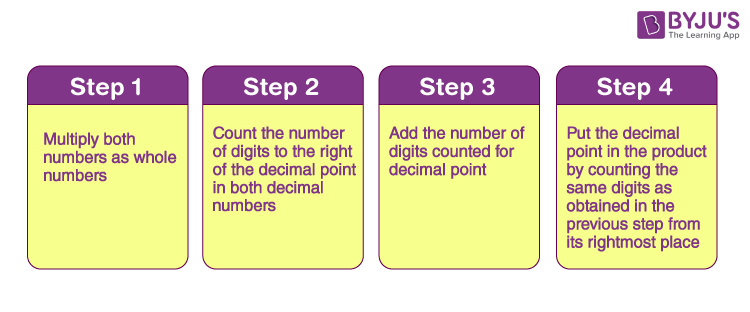Go through the example given below for understanding the above process.

Example: Multiply 923.447 by 12.6

Solution:

923.447 × 12.6

Step 1: Multiply both decimal numbers as whole numbers by removing decimal point as: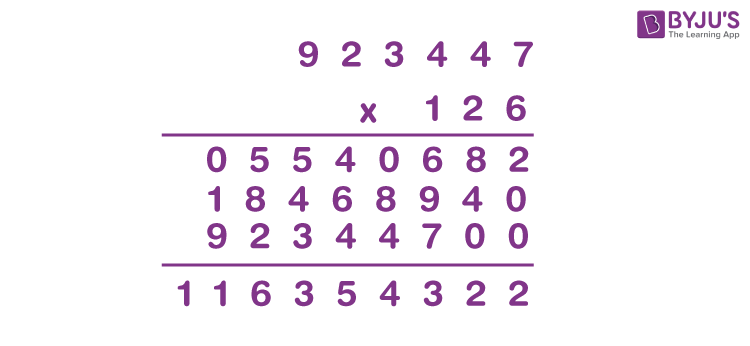Step 2: Count the number of digits to the right of the decimal point in both decimal numbers.

Thus, the number of digits after the decimal point in 923.447 = 3

And the number of decimal places in 12.6 = 1

Step 3: Add the number of digits counted for decimal point.

Total number of decimal places = 3 + 1 = 4

Step 4: Put the decimal point in the product by counting the same digits as obtained in the previous step from its rightmost place.

As the total number of decimal places is 4, the resultant product must contain the decimal point after 4 digits from the rightmost place.

Therefore, the product of the given decimals is 11635.4322.

### Multiplying Decimals Examples

Example 1: What is the product of 94.3 and 18?

Solution:

94.3 × 18

Let us write this expression by ignoring the decimal point.

943 × 18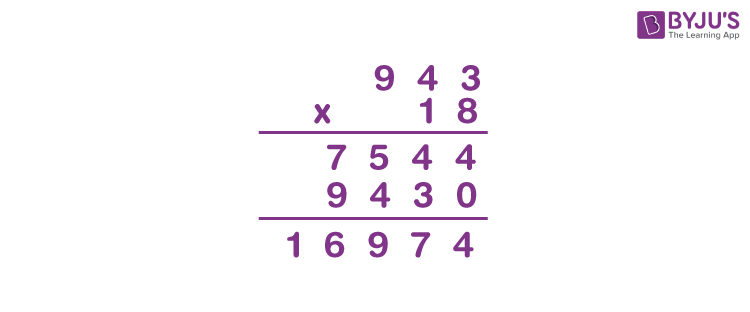Now, we have to put the decimal point in the obtained product.

Therefore, 94.3 × 18 = 169.74

Example 2: Multiply the decimal number 0.785 by 0.055.

Solution:

0.785 × 0.055

Let us write this expression by ignoring the decimal point.

785 × 55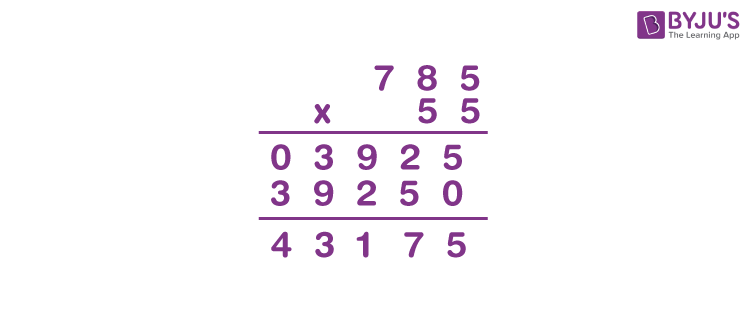Now, we have to find the total number of decimal places.

Number of decimal places in 0.785 = 3

Number of decimal places in 0.055 = 3

Thus, the total number of decimal places = 3 + 3 = 6

That means, we have to put the decimal point after six digits from the rightmost place.

Hence, the product is 0.043175.